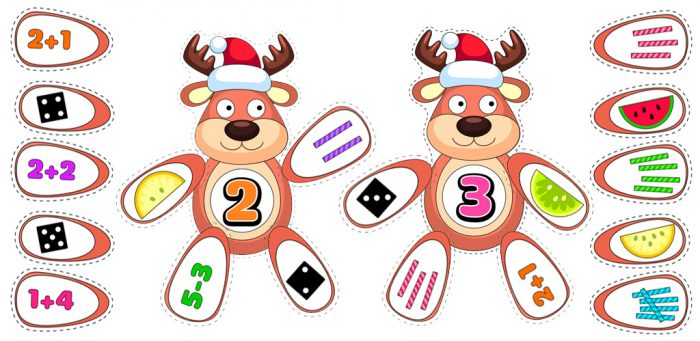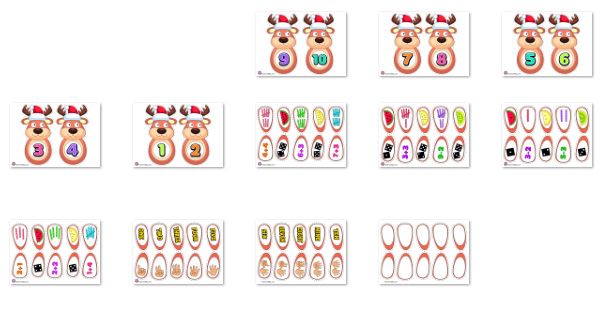# Reindeer Number Match Activity

\$2.00

12 sheets

This number comprehension activity uses Reindeer to help students understand the various ways to illustrate numbers and do math equations to determine a number.

## Description## Reindeer

### Number Match Printables

This number comprehension activity uses Reindeer to help students understand the various ways to illustrate numbers and do math equations to determine a number.

For this activity the Reindeer have a number on their torso and then students need to find the hooves that equal that number either via a symbol (such as a hand holding-up the matching fingers) or a math equation (such as how 2+1 would equal the number three).

Also, here are some blank hooves that you can write more equations in for students to use.

It is a fun way to tie-in learning about numbers with Christmas Reindeer!

### PDF file / 12 sheets